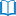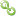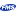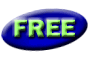Book RecommendationsLinks to Related SitesMy FMS

Thank you! Thank you! I just finished reading this document, which was part of a link in the recent Buzz newsletter. I have printed it for others to read, especially those skeptical on the powers of Access and its capabilities.

Darren D.IIF vs. If...Then...Else in VB, VBA, and VB .NET

Provided by: Molly Pell, Technical Project Manager

Visual Basic, VBA, and Visual Basic .NET support the IIF function as an alternative to the If...Then...Else statement. Although this may seem like a shortcut, IIF functions differently than If...Then...Else.

IIF must evaluate the entire statement when preparing the argument, which can lead to undesirable side effects. Consider the following code:

VBA and Visual Basic:

Public Function Divide(ByVal n1 As Integer, n2 As Integer) As Variant
Divide = IIf(n2 = 0, MsgBox("Cannot divide by 0"), MsgBox(n1 / n2))
End Function

VB.NET:

Public Function Divide(ByVal n1 As Integer, n2 As Integer) As Object
Return IIf(n2 = 0, MsgBox("Cannot divide by 0"), MsgBox(n1 / n2))
End Function

The intention of this code is to check whether the divisor is 0. If it is not 0, then we want to perform the division. If it is 0, then we want to tell the user that it cannot divide by 0.

When this function is run, however, both the True and the False expressions are executed. Regardless of the values passed, the code first shows the "Cannot divide by 0" message, and then shows a message box displaying the result. Or even worse, if the divisor is 0, the code breaks with an unhandled exception.

Resolution

Consider replacing the IIF statement with If...Then...Else. For instance, you could change the example above to:

VBA and Visual Basic:

Public Function Divide(ByVal n1 As Integer, n2 As Integer) As Variant
If n2 = 0 Then
MsgBox ("Cannot divide by 0")
Else
MsgBox (n1 / n2)
End If
End Function

VB.NET:

Public Function Divide(ByVal n1 As Integer, n2 As Integer) As Object
If n2 = 0 Then
MsgBox("Cannot divide by 0")
Else
MsgBox (n1 / n2)
End If
End Function

This tip and more detected for you with Total .NET Analyzer and Total Access Analyzer!